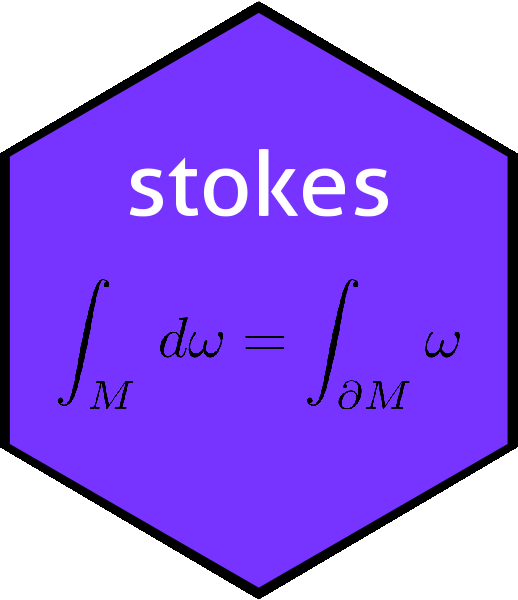# The inner() function in the stokes packageinner
## function (M)
## {
##     ktensor(spray(expand.grid(seq_len(nrow(M)), seq_len(ncol(M))),
##         c(M)))
## }
## <bytecode: 0x55abc5c71558>
## <environment: namespace:stokes>

Function inner() returns the inner product corresponding to a matrix.

The inner product of two vectors $$\mathbf{x}$$ and $$\mathbf{y}$$ is usually written $$\left\langle\mathbf{x},\mathbf{y}\right\rangle$$ or $$\mathbf{x}\cdot\mathbf{y}$$, but the most general form would be $$\mathbf{x}^TM\mathbf{y}$$ where $$M$$ is a matrix. Noting that inner products are multilinear, that is $$\left\langle\mathbf{x},a\mathbf{y}+b\mathbf{z}\right\rangle=a\left\langle\mathbf{x},\mathbf{y}\right\rangle + b\left\langle\mathbf{x},\mathbf{z}\right\rangle$$ and $$\left\langle a\mathbf{x} + b\mathbf{y},\mathbf{z}\right\rangle=a\left\langle\mathbf{x},\mathbf{z}\right\rangle + b\left\langle\mathbf{y},\mathbf{z}\right\rangle$$ we see that the inner product is indeed a multilinear map, that is, a tensor.

inner(diag(7))
## A linear map from V^2 to R with V=R^7:
##          val
##  6 6  =    1
##  7 7  =    1
##  5 5  =    1
##  3 3  =    1
##  2 2  =    1
##  4 4  =    1
##  1 1  =    1

Note how the rows of the tensor appear in arbitrary order. Verify:

x <- rnorm(7)
y <- rnorm(7)
V <- cbind(x,y)
c(as.function(inner(diag(7)))(V),sum(x*y))  # should match
##  5.503805 5.503805

A more stringent test would be to use a general matrix:

M <- matrix(rnorm(49),7,7)
f <- as.function(inner(M))
c(f(V),quad.3form(M,x,y)) # should match
##  -3.41066 -3.41066

(function emulator::quad.3form() evaluates matrix products efficiently; quad.form(M,x,y) returns $$x^TMy$$). Of course, we would expect inner() to be a homomorphism:

M1 <- matrix(rnorm(49),7,7)
M2 <- matrix(rnorm(49),7,7)
g <- as.function(inner(M1+M2))
c(g(V),quad.3form(M1+M2,x,y)) # should match
##  -5.418253 -5.418253

Now, if the matrix is symmetric the corresponding inner product should also be symmetric:

h <- as.function(inner(M1 + t(M1)))
c(h(V), h(V[,2:1]))  # should match
##  -22.52436 -22.52436

Also positive-definite matrix should return a positive quadratic form:

M3 <- crossprod(matrix(rnorm(56),8,7))  # 7x7 pos-def matrix
as.function(inner(M3))(kronecker(rnorm(7),t(c(1,1))))>0  # should be TRUE
##  TRUE

## Alternating forms

The inner product on an antisymmetric matrix should be alternating:

jj <- matrix(rpois(49,lambda=3.2),7,7)
M <- jj-t(jj) # M is antisymmetric
f <- as.function(inner(M))
c(f(V),f(V[,2:1])) # differ in sign
##   19.50013 -19.50013